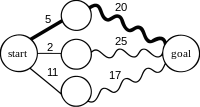# Optimal substructureFigure 1. Finding the shortest path using optimal substructure. Numbers represent the length of the path; straight lines indicate single edges, wavy lines indicate shortest paths, i.e., there might be other vertices that are not shown here.

In computer science, a problem is said to have optimal substructure if an optimal solution can be constructed from optimal solutions of its subproblems. This property is used to determine the usefulness of dynamic programming and greedy algorithms for a problem.

Typically, a greedy algorithm is used to solve a problem with optimal substructure if it can be proven by induction that this is optimal at each step. Otherwise, provided the problem exhibits overlapping subproblems as well, dynamic programming is used. If there are no appropriate greedy algorithms and the problem fails to exhibit overlapping subproblems, often a lengthy but straightforward search of the solution space is the best alternative.

In the application of dynamic programming to mathematical optimization, Richard Bellman's Principle of Optimality is based on the idea that in order to solve a dynamic optimization problem from some starting period t to some ending period T, one implicitly has to solve subproblems starting from later dates s, where t<s<T. This is an example of optimal substructure. The Principle of Optimality is used to derive the Bellman equation, which shows how the value of the problem starting from t is related to the value of the problem starting from s.

## Example

Consider finding a shortest path for travelling between two cities by car, as illustrated in Figure 1. Such an example is likely to exhibit optimal substructure. That is, if the shortest route from Seattle to Los Angeles passes through Portland and then Sacramento, then the shortest route from Portland to Los Angeles must pass through Sacramento too. That is, the problem of how to get from Portland to Los Angeles is nested inside the problem of how to get from Seattle to Los Angeles. (The wavy lines in the graph represent solutions to the subproblems.)

As an example of a problem that is unlikely to exhibit optimal substructure, consider the problem of finding the cheapest airline ticket from Buenos Aires to Moscow. Even if that ticket involves stops in Miami and then London, we can't conclude that the cheapest ticket from Miami to Moscow stops in London, because the price at which an airline sells a multi-flight trip is usually not the sum of the prices at which it would sell the individual flights in the trip.

## Definition

A slightly more formal definition of optimal substructure can be given. Let a "problem" be a collection of "alternatives", and let each alternative have an associated cost, c(a). The task is to find a set of alternatives that minimizes c(a). Suppose that the alternatives can be partitioned into subsets, i.e. each alternative belongs to only one subset. Suppose each subset has its own cost function. The minima of each of these cost functions can be found, as can the minima of the global cost function, restricted to the same subsets. If these minima match for each subset, then it's almost obvious that a global minimum can be picked not out of the full set of alternatives, but out of only the set that consists of the minima of the smaller, local cost functions we have defined. If minimizing the local functions is a problem of "lower order", and (specifically) if, after a finite number of these reductions, the problem becomes trivial, then the problem has an optimal substructure.

## Problems without optimal substructure

• Longest path problem